By Long Luo

PID 算法 是自动控制领域中很重要的算法。

# Simple PID Controller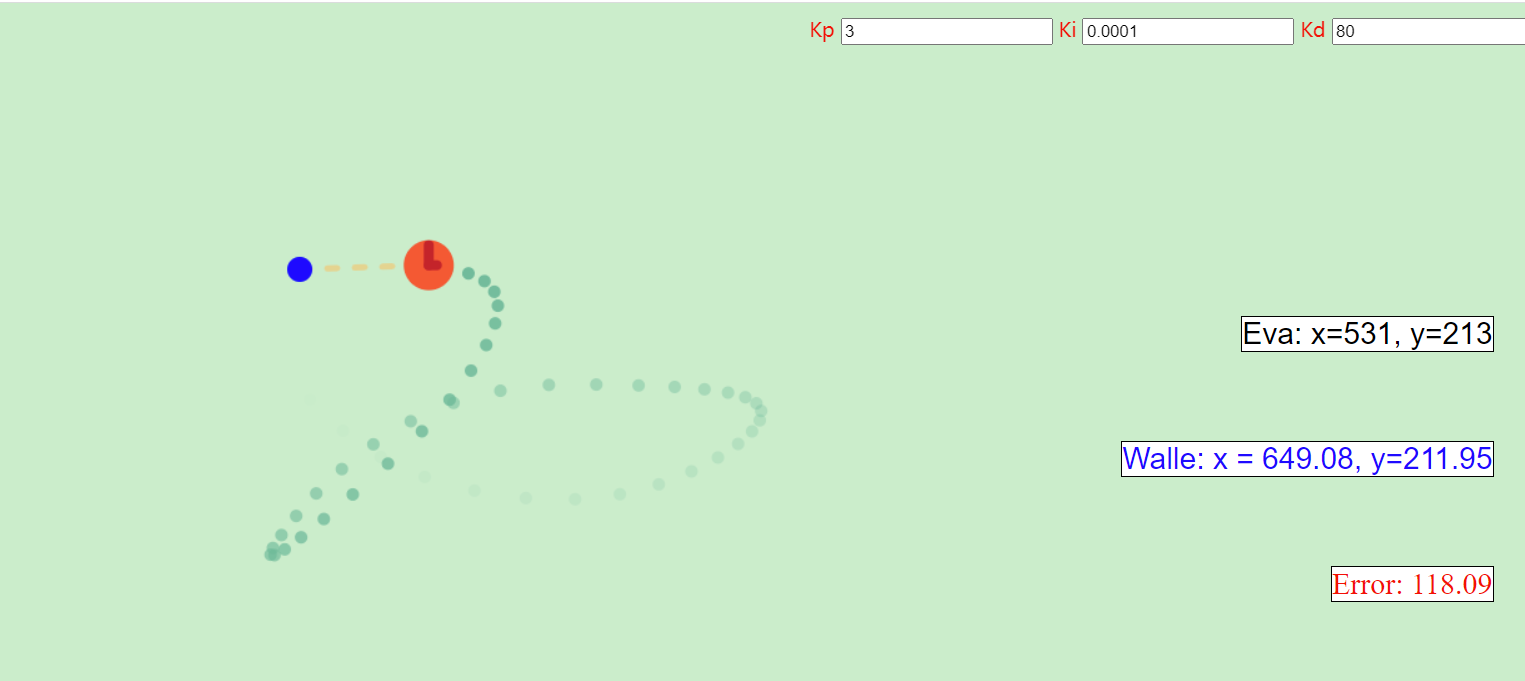1. 增加机器人速度 $v$ 及加速度 $a$ 显示；
2. 增加 2 个图表展示 PID X 轴方向及 Y 轴方向的 P、I、D $3$ 个分量随时间变化显示；
3. 之前代码将时间及速度固定了，但这不符合实际，增加随 $dt$ 变化积分和微分项；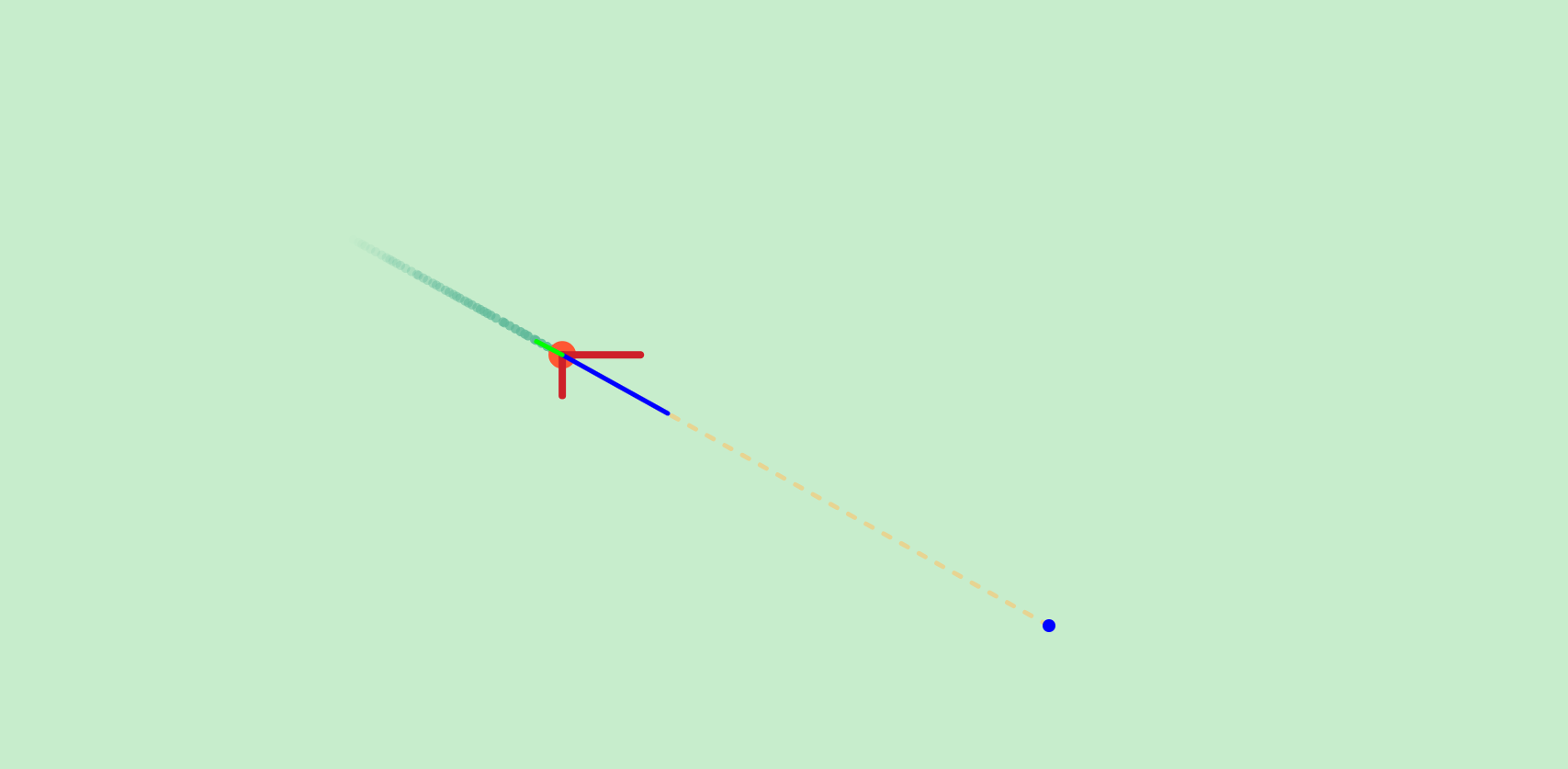By Long Luo

Kalman Filter 是贝叶斯滤波器的一种特殊实现。

# Kalman Filter 1D | 一维卡尔曼滤波器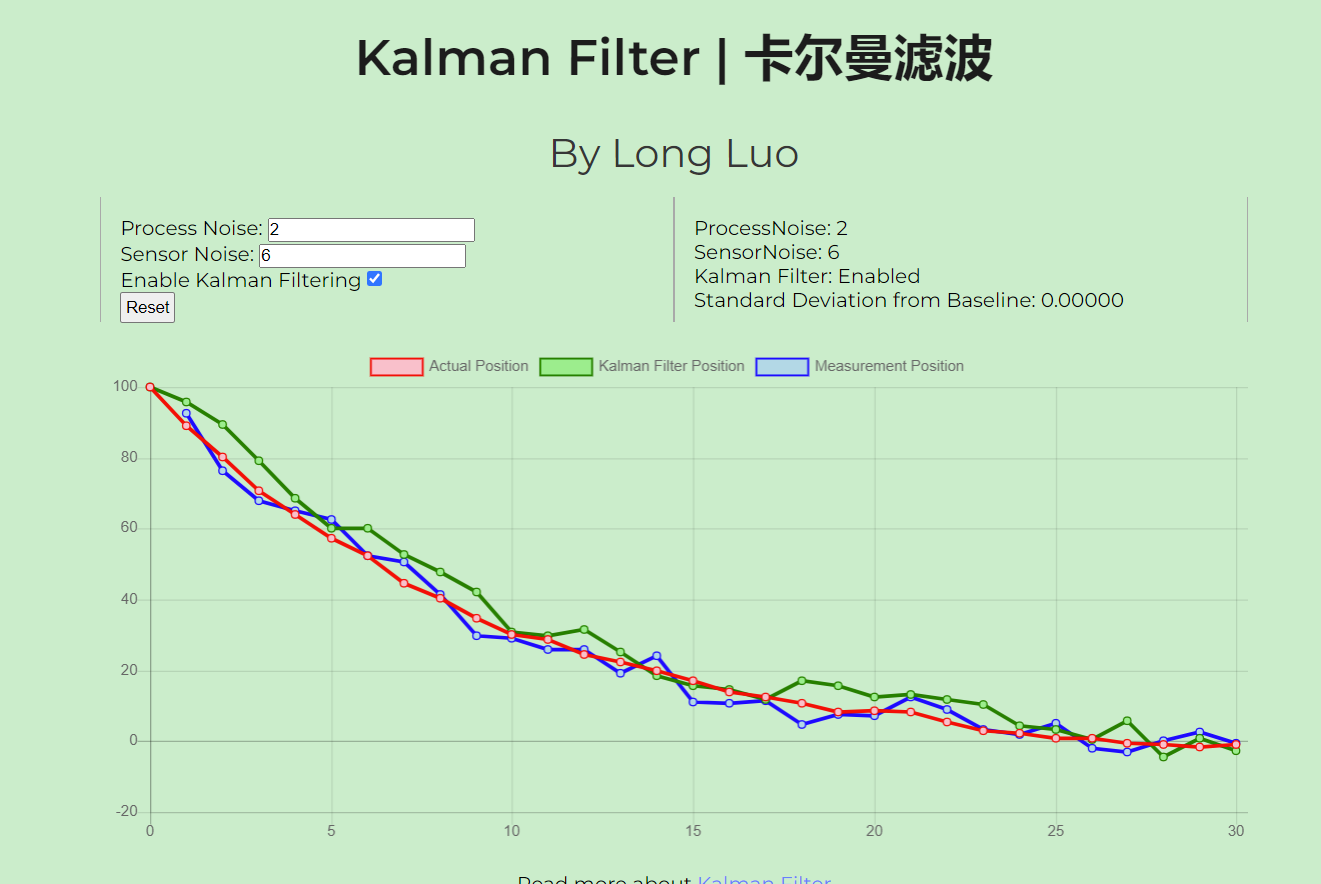By Long Luo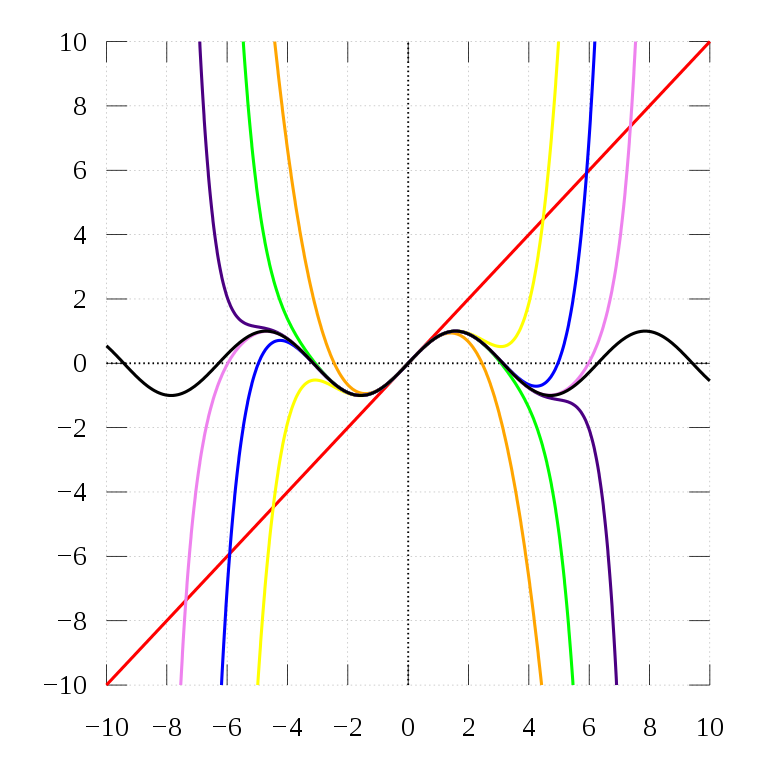$f(x) = \sum _{n=0}^{\infty}{\frac{f^{(n)}(a)}{n!}}(x-a)^{n} = f(a) + {\frac {f'(a)}{1!}}(x - a) + {\frac {f''(a)}{2!}}(x - a)^{2} + {\frac {f'''(a)}{3!}}(x - a)^{3} + \cdots$

# 几何级数 Geometric series

$\frac{1}{1 - x} = \sum _{n=0}^{\infty}x^{n} = 1 + x + x^{2} + \cdots + x^{n}$

$-x$ 代入 $x$ 上式，则：

$\frac{1}{1 + x} = \sum _{n=0}^{\infty}(-1)^nx^{n} = 1 - x + x^{2} - x^3 + \cdots + (-1)^n x^{n}$

$x^2$ 替代 $x$ , 由于 $\arctan x = \int_{0}^{x} \frac{1}{1 + x^2} \mathrm{d}x$ ，对于 $-1 \le x \le 1, x \neq \pm i$

$\arctan x = \sum _{n=0}^{\infty }{\frac {(-1)^{n}}{2n + 1}}x^{2n + 1} = x - {\frac {x^3}{3}} + {\frac {x^5}{5}} - \cdots + \frac{(-1)^n}{2n + 1}x^{2n + 1}$

\begin{aligned} \frac {1}{(1-x)^2} &= \sum _{n=1}^{\infty }n x^{n-1} \\ &= 1 + 2x + 3x^2 + \cdots + n x^{n-1} \end{aligned}

$\frac{1}{(1 - x)^3} = \frac{1}{2} (\frac{1}{(1 - x)^2})'$ ，则有：

$\frac {1}{(1 - x)^3} = \sum _{n=2}^{\infty }{\frac {n(n - 1)}{2}}x^{n - 2}$

# 指数函数 Exponent function

$e^x = \sum _{n=0}^{\infty }{\frac{x^n}{n!}} = 1 + x + {\frac{x^2}{2!}} + {\frac {x^3}{3!}} + \cdots + {\frac{x^n}{n!}}$

\begin{aligned} (e^x)' &= (\frac{1}{0!}+\frac{x}{1!}+\frac{x^2}{2!}+\frac{x^3}{3!}+\cdots)' \\ e^x &= 0+1+\frac{x}{1}+\frac{x^2}{2!}+\frac{x^3}{3!}\cdots \\ &= 1+x+\frac{x^2}{2!}+\frac{x^3}{3!}+\cdots \end{aligned}

\begin{aligned} a^x &= e^{x \ln a} \\ &= 1 + x \ln a + \frac{(x \ln a)^2}{2!} + \frac{(x \ln a)^3}{3!} + \cdots + \frac{(x \ln a)^n}{n!} \\ \end{aligned}

By Long Luo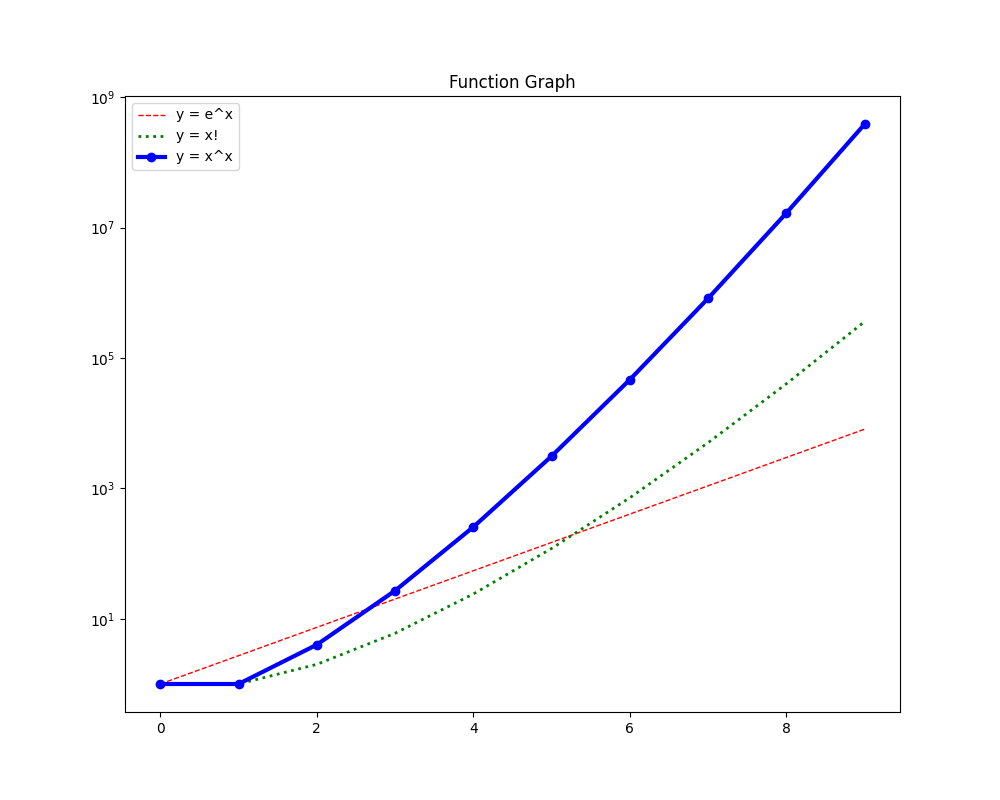# 对数放缩法 (Zoom)

$A = \log_{2}{(2^{100!})} = 100!$

$B = \log_{2}{2^{100}!}= \log_{2}{2^{100}} + \log_{2}{(2^{100} - 1)} + \dots + 1 + 0$

$B < 100 \cdot 2^{100} < 128 \cdot 2^{100} = 2^7 \cdot 2^{100} = 2^{107}$

$100!$ 至少有 $(100 - 64 + 1) = 37$ 项是大于等于 $64 = 2^6$ ，也就是 $(2^6)^{37}=2^{222}$

# 斯特林公式 (Stirling’s Approximation)

$n! \sim \sqrt{2 \pi n}(\frac{n}{e})^n = \sqrt{2 \pi} n^{\frac{n + 1}{2}}e^{-n}$

$\ln{n!} \sim \frac{1}{2} ln(2 \pi) + (n+\frac{1}{2}) ln(n) - n$

$\ln(n!) \sim n(ln(n) - 1)$

$\begin{array}{l} A = ln(2^{100!}) = 100! ln(2) \\ B = ln(2^{100}!) \sim 2^{100}(ln(2^{100}) - 1) = 2^{100}(100 ln(2) - 1) \end{array}$

$2^{100!} \gg 2^{100}!$

By Long Luo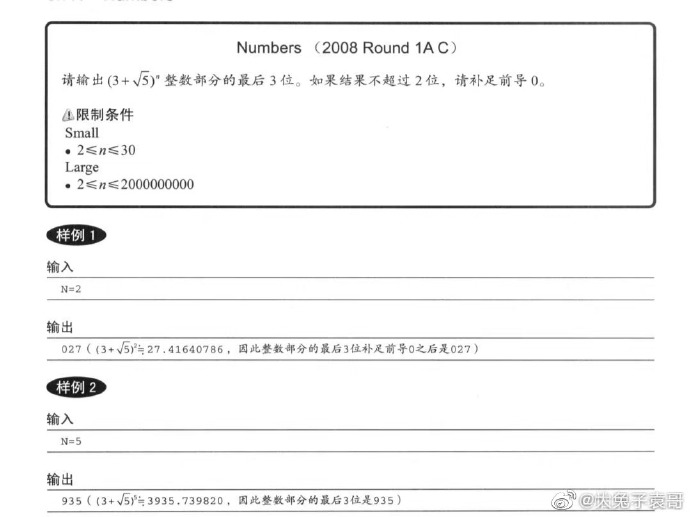$y = (3+ \sqrt{5})^n$ ，两边同取对数，$\log_{10}{y} = n \log_{10}{(3+\sqrt5)}$$y = 10^{n\log_{10}{5.23607}}$$lg5 \approx 0.7$ ，所以 $y \approx 10^{0.7n}$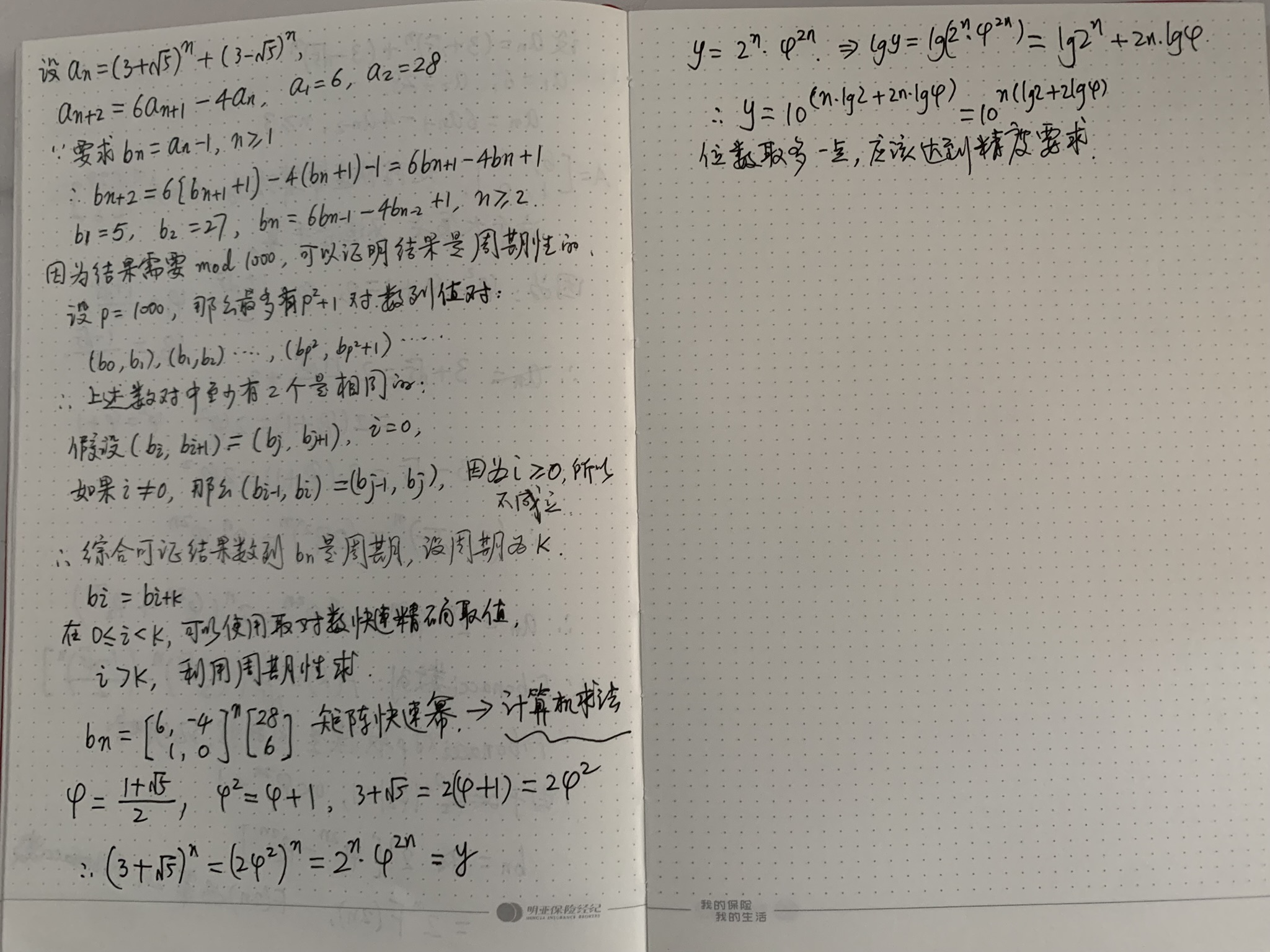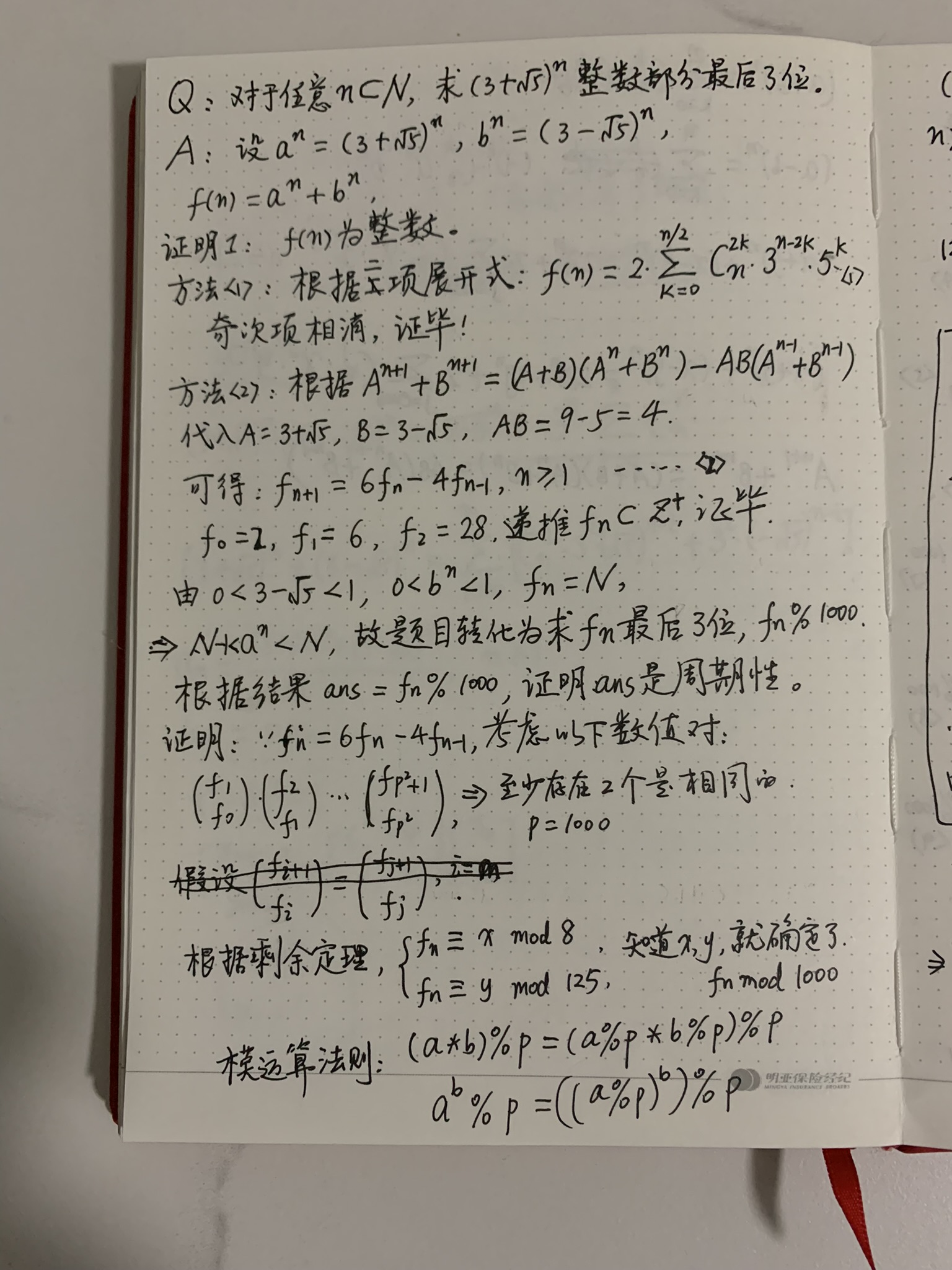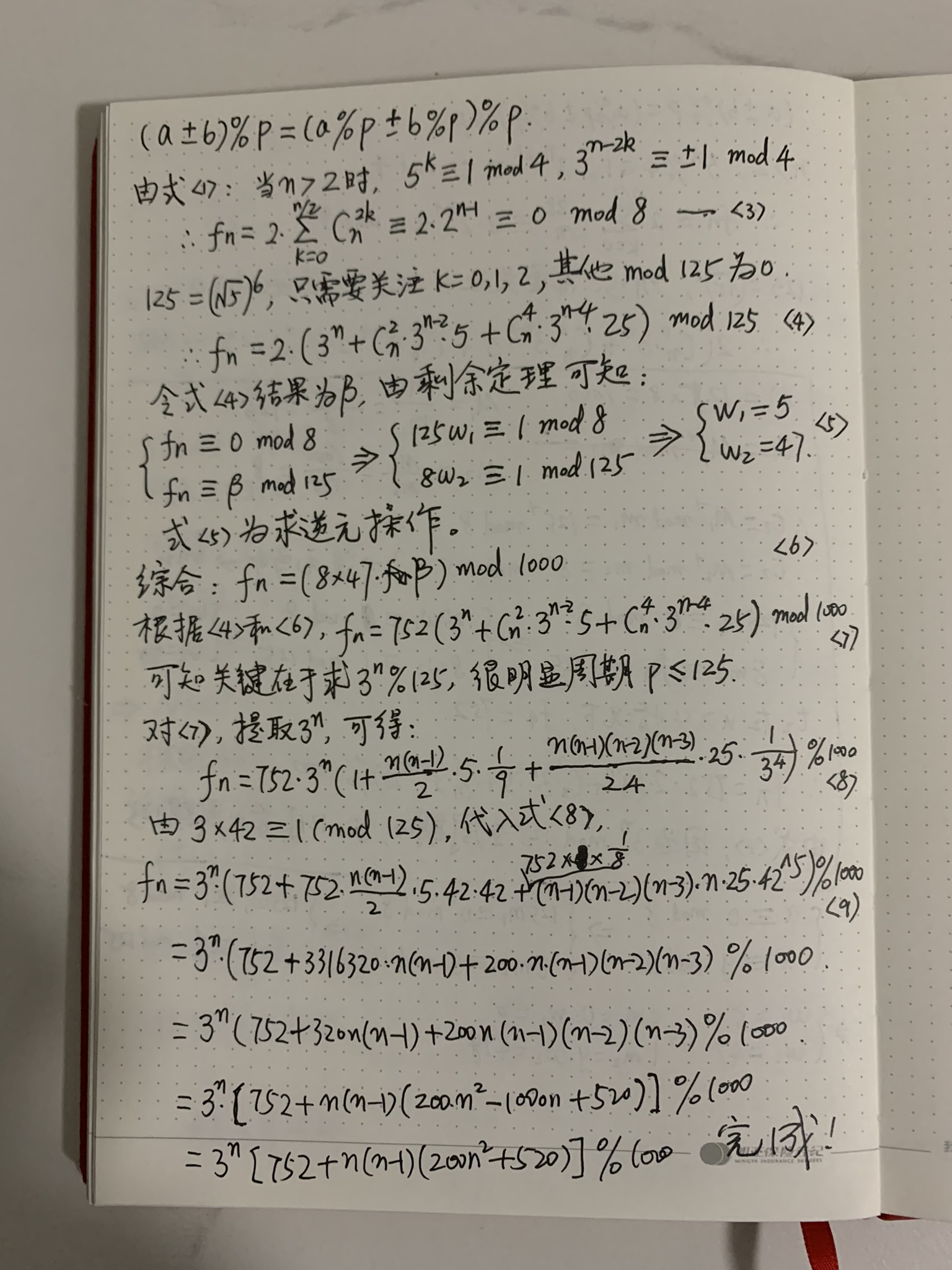0%